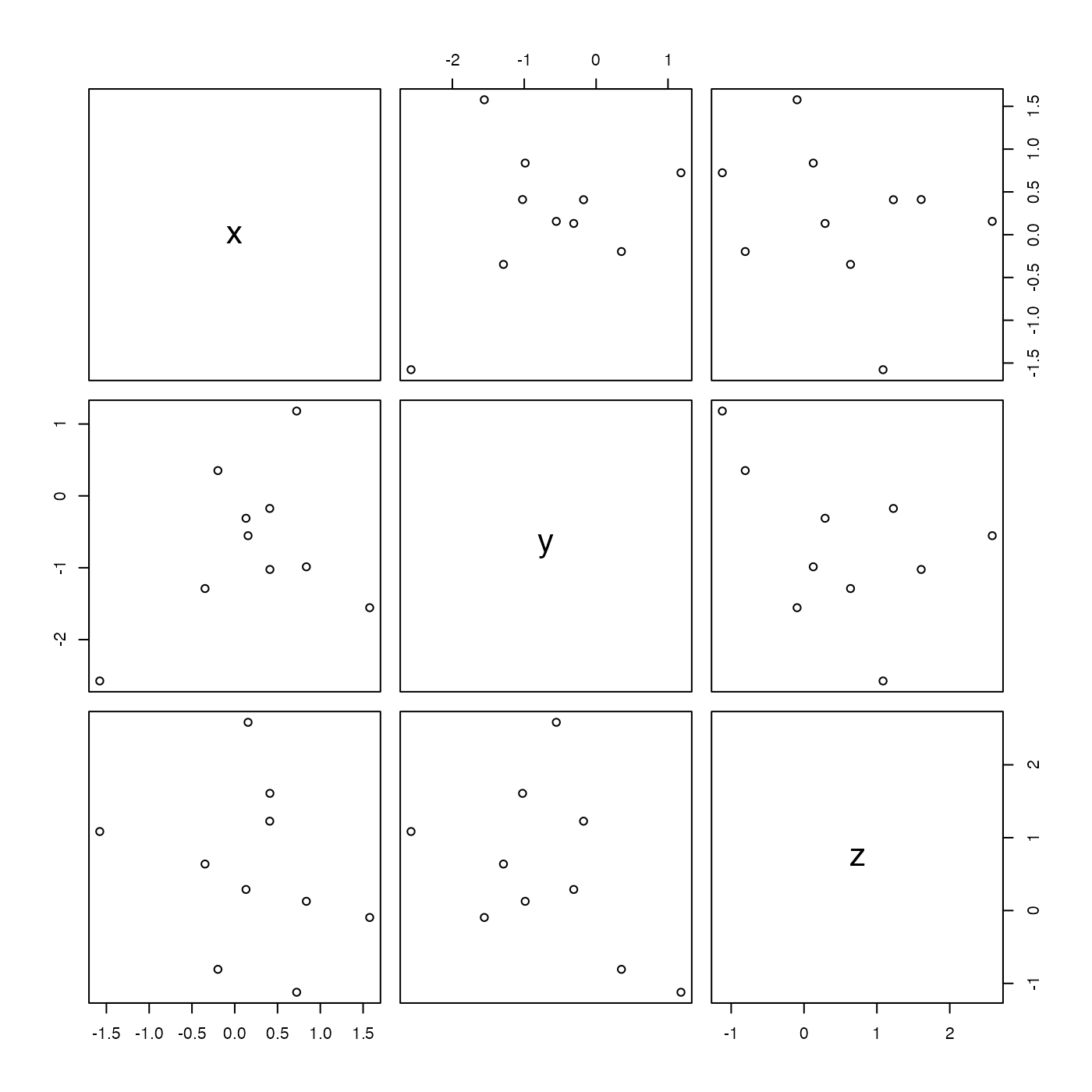This creates a pairs() plot of the elements in the data slot, if there are more than 2 elements there, or a simple xy plot if 2 elements, or a histogram if 1 element.

# S4 method for oce
plot(x, y, ...)

## Arguments

x a basic oce object, but not from any subclass that derive from this base, because subclasses have their own plot methods, e.g. calling plot() on a ctd object dispatches to plot,ctd-method(). Ignored; only present here because S4 object for generic plot need to have a second parameter before the ... parameter. Passed to hist(), plot(), or to pairs(), according to whichever does the plotting.

## Examples

library(oce)
o <- new("oce")
o <- oceSetData(o, 'x', rnorm(10))
o <- oceSetData(o, 'y', rnorm(10))
o <- oceSetData(o, 'z', rnorm(10))
plot(o)